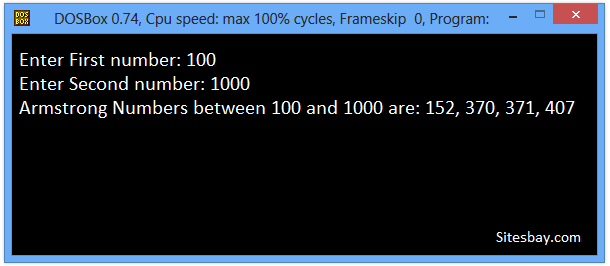# Print Armstrong Number Between Two Intervals in C++

## C++ Program to Display Armstrong Number Between Two Intervals

An Armstrong Number is a number where the sum of the digits raised to the power of total number of digits is equal to the number. Armstrong numbers are as follows-

## Armstrong Number

```3 = 3^1
153 = 1^3 + 5^3 + 3^3 = 1 + 125 + 27 = 153
407 = 4^3 + 0^3 + 7^3 = 64 +0 + 343 = 407
1634 = 1^4 + 6^4 + 3^4 + 4^4 = 1 + 1296 + 81 + 256 = 1634
```

To understand below example, you have must knowledge of following C++ programming topics; If Else Statement in C++ and For Loop in C++. In below code we use pow() function for calculate power of any number number.

In below code we receive two value from user first is lower bound and second is upper bound.

## Print Armstrong Number Between Two Intervals in C++

```#include<iostream.h>
#include<conio.h>
#include<math.h>

void main()
{
int first,second,lowerbound, upperbound, digitSum, temp, remainderNum, digitNum ;
lowerbound=1,upperbound=1;
clrscr();

cout<<"Enter Frist Number: ";
cin>>lowerbound;
if(lowerbound>100)
{
cout>>"Please Enter more than 100 first number";
}
else
{
cout<<"Enter Second Number: ";
cin>>upperbound;
cout<<"Armstrong Numbers between "<<lowerbound<<" and "<<upperbound<<" are: ";
for(int num = lowerbound; num <= upperbound; num++) {
temp = num;
digitNum = 0;
while (temp != 0) {
digitNum++;
temp = temp/10;
}
temp = num;
digitSum = 0;
while (temp != 0) {
remainderNum = temp%10;
digitSum = digitSum + pow(remainderNum, digitNum);
temp = temp/10;
}
if (num == digitSum)
cout<<num<<" ";
}
}
getch();
}
```

## Output

```Enter First number: 100
Enter Second number: 1000
Armstrong Numbers between 100 and 1000 are: 152, 370, 371, 407
```

## Output

```Enter First number: 15
Please Enter more than 100 first number
```## Pure VPN Privide Lowest Price VPN Just @ \$1.65. Per Month with Non Detected IP Lowest Price Non Detected IP VPN